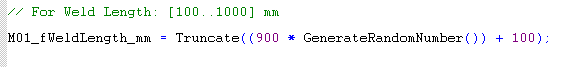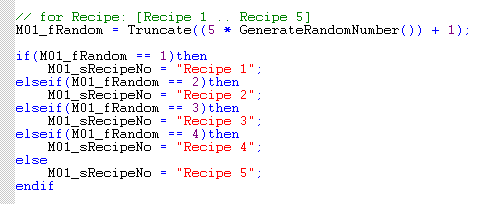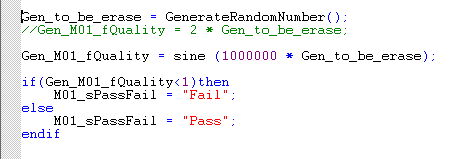# Generate random return less than 0.01 always

Hello,

I desired to implement random: Pass or Fail, code.
So I generate random and store in on fRand.
fRand = GenerateRandomNumber();
if(fRand<0.5)then
//Pass
else
//Fail
Endif

But fRand always return less than 0.01. — this is very weird, I know this is still random, but is still not proportion.

Any other way to produce random number?

How about I generate a high frequency sine wave, and generate a delay statement,
where ever the position when delay stop, that would be the value…

LOL. This will make my life more difficult.

Sorry to ans my own question… wth.

This is the code I use to produce random numbers that have some solid offset.
You can mess with the offset numbers and get them to where you need them.

``````random_number_1 = (((GenerateRandomNumber() * 11.0)) + 22.9 * 2.1685);
random_number_2 = (((GenerateRandomNumber() - 0.5) / 1.2) + 38.9 * 0.1685);``````

Are you calling SeedRandomNumber() in your strategy?

Hi Philip, I have few Generate Random command on other charts that are running concurrently.
But this one is interesting. It always generate less around 0.00nn

Yes, I tried to call SeedRandomNumber(), but results did not change.

I will try to use fRandom = sine (GenerateRandomNumber()); I am still thinking how the formula should look like to increase frequency (shorten the period).

Some sine wave code to get you started (we use it in our demos).

`````` Wave_TimeMs = Wave_UpTimer * 1000;

Wave_SineOf = (Wave_TimeMs % (Wave_Cfg_PeriodSec*1000)) / (1000.0 * Wave_Cfg_PeriodSec) * 2.0 * 3.14159;

Wave_SineValue = Sine(Wave_SineOf);

Wave_CurrentValue = Wave_Cfg_Base + Wave_Cfg_Delta * Wave_SineValue;``````

So the others work properly and this one doesn’t? Are you sure fRand is not getting changed in another chart? Something isn’t right. Can you run just his code and disable the rest of your charts and see if it works?

Look At this:
for random length, i expect it to be 100 to 1000.for random recipe:for pass and fail:The Gen Value is return from GenerateRandomNumber Command. It always stays on 0.00nn

Looks like the random recipe is working… others not.
I might use the sine function.
But GenerateRandomNumber is very weird, maybe because I am using softpac installed on Azure Cloud Virtual Windows?

Can you do something simple like this:

``````for n=0 to 49 step 1
floattable[n] = GenerateRandomNumber();
next
``````

and see what comes back?

Hi Philip,

Please observer first index. - I ran this command between 80 to 120 sec, random in between.

I think what is happening, the first “generation” is always 0.00nn area…
on 3rd generation onwards, it becomes random.

This is why, Random Recipe code is random, from observation… because Random Recipe code is generated after few other random variables…

Looks like you found a bug.

Which Version of SoftPAC are you running? I am getting the same first low number result on 9.5g, but it looks like 10.0d works okay.

Softpac version R10.0f

Let me submit this to support team.

Thank you very much.